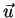Next: Relative Boundary Orientation Up: Description of Three Dimensional Previous: Boundary Length

# Parallel Boundaries

A distinctive surface feature is the presence of parallel boundary sections. Hence, one potential description for surfaces is the number of groups of parallel boundaries. In this context, parallel means in three dimensions, and requires:

• vectors between endpoints to be parallel, and
• direction of arc curvature to be parallel.

The endpoint vector calculation is trivial given the three dimensional data. The direction vectors are thedefined in the previous section, so the second test is also easy. The results for the test image are given in Table 6.3. No errors occurred.

Bob Fisher 2004-02-26